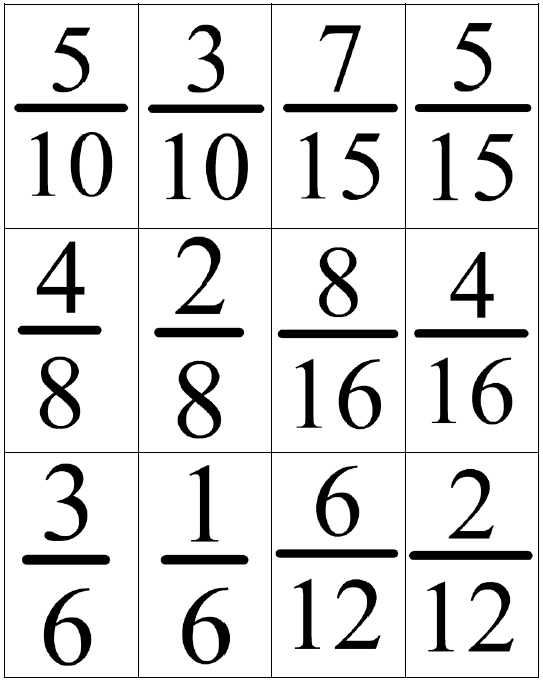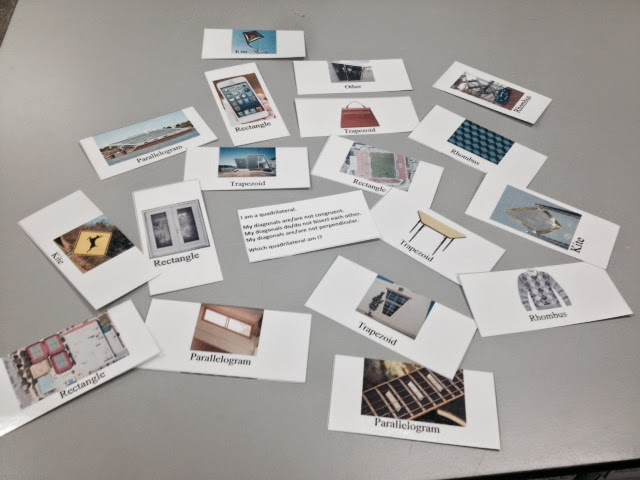## Friday, 27 June 2014

### Sort Students into Groups using Equivalent FractionsIf you want a quick activity that you can use to randomly put students into groups of four, look no further. This activity uses fractions with common denominators and then equivalent fractions to sort students into groups. This could be used with any class where students can add fractions and compare equivalent factions.
In each row are two sets of fractions with common denominators. When you add each pair (with the same denominator) they will simplify to the same fraction
• Grade 7 Number Sense & Numeration
• Grade 8 Number Sense & Numeration (review)
• MPM1D
• MFM1P
1. Choose a fraction and  find your partner (the  person who has the same  common denominator). Introduce yourself. He  or she is your team  member.
3. Find the other team  with the same simplified  fraction. Introduce  yourselves. This is your group of four
• None yet
• Choosing Groups with Fractions Cards (doc) (pdf)

Did you use this activity? Do you have a way to make it better? If so tell us in the comment section. ThanksMade popular on The Ellen Show, Heads Up is a game where one person holds a card on their forehead (facing their opponent) and the second person has to give hints about what is on the card (without using any of the words on the card). The first person uses the clues to try to guess what is on their forehead.
In grade 8 we have an expectation that requires students to classify quadrilaterals based on characteristics of the diagonals. This activity does a good job of consolidating that knowledge in a fun game.

• Grade 8 Geometry and Spatial Sense - sort and classify quadrilaterals by geometric properties, including those based on diagonals, through investigation using a variety of tools. Can also relate to : investigate and describe applications of geometric properties in the real world.
• MPM1D (for review of polygon properties)• One set of Quadrilateral Picture cards per pair of students
• One Quadrilateral Prompt card per pair of students1. Give each pair of students one set of cards (18 picture cards and one prompt card)
2. One student is the "guesser" and the other gives the clues.
3. The guesser randomly selects a picture card, without looking at it, and places it on their forehead.
4. The other person gives clues about the properties of the quadrilaterals seen on the picture card. They should use clues relating to the diagonals (to hit the expectation) and may use the prompt card to help remind them of possible clues they can give. Note that by focussing the clues on the diagonals, this requires both the guesser and clue giver to know about their properties relating to quads. Note that we have included an image on each card to connect to the real world. However, we want the clues to be about the diagonals not about the pictures (ie we don't want students to say "its a football field" as a clue)
5. Once the guesser guesses correctly, they can choose another card and play again or the two students can switch roles.None yet but here is a clip of it happening on The Ellen Show

• Quadrilateral Heads Up Prompt Card (doc) (pdf) There are 6 cards on this sheet but only one is needed per group.

Did you use this activity? Do you have a way to make it better? If so tell us in the comment section. Thanks

### Algebra Tile Musical DesksWhen you bring out algebra tiles students tend to automatically create designs. So why not include that in your lesson?  In this activity students create a design using algebra tiles and simplify the design using the zero principle.MPM1D MFM1P

• Algebra tiles
• Music
• Whiteboards and markers (optional)
1. Students create a design using algebra tiles.
2. Play music and have students circulate.
3. When the music stops students must sit at a desk and simplify another students design using the zero principle.
4. Students write the resulting polynomial on the whiteboard and identify as a monomial, binomial or trinomial.
5. Repeat the same activity at the new desk or as an extension you could ask them to create a design that simplifies to a particular polynomial expresssion.  (eg.  x2 - 3x + 4)

• None
Did you use this activity? Do you have a way to make it better? If so tell us in the comment section. Thanks

## Tuesday, 24 June 2014

### Linear Scavenger HuntThe linear scavenger hunt is an activity where there are sets of four representations of linear relations on cards (graphical, tabular, algebraic and word problem) that students have to search for and match.

• MPM1D, MFM1P, MFM2P
• determine values of a linear relation by using a table of values, by using the equation of the relation, and by interpolating or extrapolating from the graph of the relation
• determine other representations of a linear relation, given one representation
• describe the meaning of the slope and y-intercept for a linear relation arising from a realistic situation
• determine the equation of a line from information about the line
• Linear Scavenger Hunt Cards (printed 1 sided)
• Linear Scavenger Hunt Question Cards (printed back to back)
• Tape/stick tack
• Beach Ball (optional)
• Whiteboard markers (optional)
1. Each group of 3 students gets 1 representation of a linear relationship  - either a story, equation, table or graph.  Students will complete a scavenger hunt by roaming around the room to find the other 3 representations of their linear relationship. The other three cards in each set are randomly distributed around the class before hand.
2. Once all four cards are together, each group must fill in missing information on the graph (title and axes labels) and in the table (column titles and table values -  CAUTION:  yellow rows are out of step in the pattern). Teacher can check missing information quickly using reference card.
3. Each group will tape their equation in the middle of their desks.
4. Each group will now tape their story to the beach ball.  Students will throw the ball and each group will select a card (not their own).  That group must now find the equation of the new story they got off the beach ball.  One member will then circulate to check if the equation is correct with the original group for their new story and return it to that group.
5. Repeat step 4 with the tables.
6. Repeat step 4 with the graphs.
7. Each group should now have the original 4 representations of their linear relationship.  Students will now answer 5 questions related to their relationship found on the Question Cards.
Other possible uses
• give a story or equation to each student and have them find their partner
• give each group of 3 an equation and have them describe several situations that could be modelled by the given linear equation.
• give students a card and have them identify as a direct or partial variation
• give a student a table or graph - have them find the rate and explain its meaning

The video, below is only visible in the WECDSB domain. That is, only teachers in our school board can see the video if they are logged into their MyTools2Go accounts.

• Linear Scavenger Hunt Cards (doc) (pdf)
• Linear Scavenger Hunt Question Cards (doc) (pdf)
• Note that if you print these out on card stock and laminate them, students can use whiteboard markers or whiteboard crayons to do their work on them and can be reused.
Did you use this activity? Do you have a way to make it better? If so tell us in the comment section. Thanks